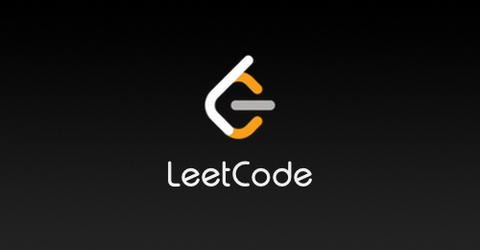update. 评论区提示这个算法在02年的文章中介绍related results的部分已经被提到过了。所以果然又是重新发明。那么好奇众数的那部分是新的么...？

(原来那个网页的标题叫这个)

1. 线段树。-(这里前一个是预处理复杂度，后一个是单次询问复杂度)，或者-(比如在中有提到。现在好像有的人把这个变种叫作猫树)。

2. ST表。-3. RMQ转LCA，离线tarjan LCA。。(为了方便，这里假设询问数同阶)

update. 最近学到了怎么把tarjan LCA做到，可以看这里。但目测不好写。

4. 离线并查集。。本质和上一个类似但不需要显式地和LCA扯上关系。

5. RMQ转LCA再转±1RMQ。-。这是大家熟知的那个理论最好的做法，但是常数比较大。

6. RMQ转LCA，然后用LCA的Schieber Vishkin algorithm(可以在这里看到相关介绍)。-。历史上是先有的这个做法，然后出现了方法5，大家认为方法5更简单

const int N=100105,D_max=18,L=27,inf=1<<30;
int f[N/L][D_max],M[N/L],a[N+L],stack[L+1],n,q,D;
struct node{
int state[L+1],*a;
int Qmin(int x,int y){return a[x+__builtin_ctz(state[y]>>x)];}
void init(int *_a){
int top=0;a=_a;
for (int i=1;i<=L;++i){
state[i]=state[i-1];
while (top&&a[i]<=a[stack[top]])state[i]-=1<<stack[top],--top;
stack[++top]=i;state[i]+=1<<i;
}
}
}c[N/L];
void build(){
int nn=n/L;M=-1;
for (int i=1;i<=nn;++i){
f[i]=inf;for (int j=1;j<=L;++j)f[i]=min(f[i],a[(i-1)*L+j]);
}
for (int i=1;i<=nn;++i)M[i]=!(i&(i-1))?M[i-1]+1:M[i-1];
for (int j=1;j<=D;++j)
for (int i=1;i<=nn-(1<<j)+1;++i)f[i][j]=min(f[i][j-1],f[i+(1<<(j-1))][j-1]);
for (int i=1;i<=nn+1;++i)c[i].init(a+(i-1)*L);
}
inline int Qmin_ST(int x,int y){
int z=M[y-x+1];return min(f[x][z],f[y-(1<<z)+1][z]);
}
inline int Qmin(int x,int y){
int xx=(x-1)/L+1,yy=(y-1)/L+1,res;
if (xx+1<=yy-1)res=Qmin_ST(xx+1,yy-1);else res=inf;
if (xx==yy)res=min(res,c[xx].Qmin(x-(xx-1)*L,y-(yy-1)*L));
else res=min(res,c[xx].Qmin(x-(xx-1)*L,L)),res=min(res,c[yy].Qmin(1,y-(yy-1)*L));
return res;
}


(交BZOJ1699的完整代码可以看这里。)

update:

## 静态区间众数询问

Loading...leetcode.comp.s. 这里比一般半群的情况复杂度更好的原因是，当询问区间足够小的时候我们可以根据问题的特殊性质直接做到。这个做法对最大子段和不work的原因是虽然当足够小时本质不同的块数可以不超过，但是不容易在的时间内识别出每块属于哪种本质不同的情况。

Lemma 1. 如果数在整个数组中一共出现了次，那么把所有存在严格众数且为的区间拿出来取并集，并集最多覆盖了个数组中的元素。

Proof. 考虑那个(大家或许见过的)求区间并的贪心算法，归纳假设是已经求出了并集在中的部分，每次在未被当前并集完整包含的区间中找到左端点，右端点最大的一个区间，和当前的并集取并。如果找不到左端点的区间则取左端点最小(且右端点最大)的区间。这样我们可以用一部分区间的并表示并集，且每个元素最多被覆盖2次。接下来令表示数组的第个元素是否为，用线性规划写一系列不等式就能得证。

Lemma 2. 我们可以在的时间内求出这个并集。

Algorithm. 不妨假设所有数的出现位置已经按顺序存在一个数组中。对于每个下标，我们希望计算是否在并集中，这当且仅当存在，即(为了简单，这里我们忽略取整的细节)。那么我们只需要维护一下的前后缀min/max就行。可以发现有意义的最多只有个。

References:

 Yao A C. Space-time tradeoff for answering range queries[C]//Proceedings of the fourteenth annual ACM symposium on Theory of computing. ACM, 1982: 128-136.

 Alon N, Schieber B. Optimal preprocessing for answering on-line product queries[M]. Tel-Aviv University. The Moise and Frida Eskenasy Institute of Computer Sciences, 1987.

 Chen K Y, Chao K M. On the range maximum-sum segment query problem[C]//International Symposium on Algorithms and Computation. Springer, Berlin, Heidelberg, 2004: 294-305.

 Durocher S, He M, Munro J I, et al. Range majority in constant time and linear space[C]//International Colloquium on Automata, Languages, and Programming. Springer, Berlin, Heidelberg, 2011: 244-255.

 Yuan H, Atallah M J. Data structures for range minimum queries in multidimensional arrays[C]//Proceedings of the twenty-first annual ACM-SIAM symposium on Discrete Algorithms. Society for Industrial and Applied Mathematics, 2010: 150-160.

 Alstrup S, Gavoille C, Kaplan H, et al. Nearest common ancestors: a survey and a new distributed algorithm[C]//Proceedings of the fourteenth annual ACM symposium on Parallel algorithms and architectures. ACM, 2002: 258-264.

 Navarro G, Thankachan S V. Optimal encodings for range majority queries[J]. Algorithmica, 2016, 74(3): 1082-1098.

 Elmasry A, He M, Munro J I, et al. Dynamic range majority data structures[C]//International Symposium on Algorithms and Computation. Springer, Berlin, Heidelberg, 2011: 150-159.